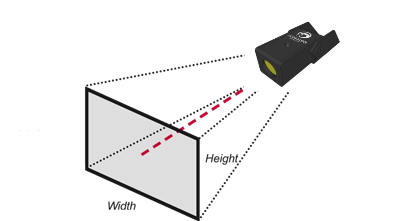LEDbar Calculator

Given the light beam angle and the distance to the target, the maximum and minimum field of view is calculated.

Scorpion Stinger LEDbar Calculator

Enter the horizontal and vertical angle, the minimum and maximum distance to the target and press the calculate button.

 Scorpion Stinger LEDbar Calculator Horizontal angle : degrees Vertical angle : degrees Minimum Distance : Feet Meters Inches mm Maximum Distance : Feet Meters Inches mmCalculated Results Width minimum distance : Height minimum distance : Width maximum distance : Height maximum distance :
2D Lens calculator

Calculate the lenses to use in your Scorpion Stinger or Venom Camera with this 2D lens calculator. The lens size is calculated based on the needed field of view; the distance from the camera to the target and the height and width of the area of interest.

 2D Lens calculator3D Lens calculator

Calculate the lenses to use in your Scorpion 3D Stinger or Venom Camera with this 3D lens calculator. The lens size is calculated based on the needed 3D field of view; the maximum and minimum distance to the target and the height and width of the area of interest.

 3D Lens calculator